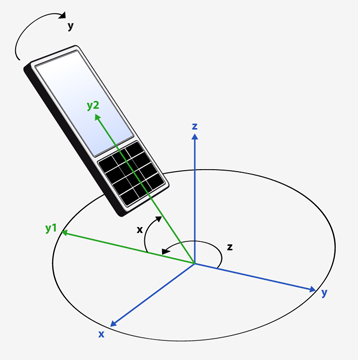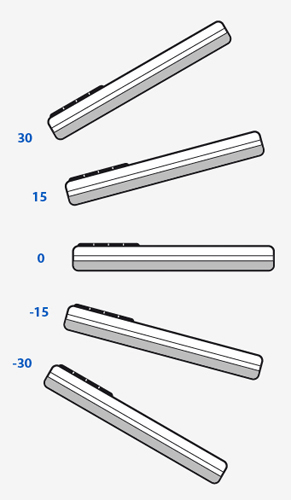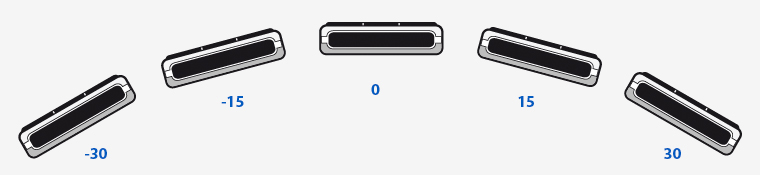This class was introduced in Qt 5.1.

## Properties

• x : const qreal
• y : const qreal
• z : const qreal

## Public Functions

 void setFromEuler(qreal x, qreal y, qreal z) qreal x() const qreal y() const qreal z() const

## Detailed Description

The rotation reading contains 3 angles, measured in degrees that define the orientation of the device in three-dimensional space. These angles are similar to yaw, pitch and roll but are defined using only right hand rotation with axes as defined by the right hand cartesian coordinate system.The three angles are applied to the device in the following order.

• Right-handed rotation z (-180, 180]. Starting from the y-axis and incrementing in the counter-clockwise direction.
• Right-handed rotation x [-90, 90]. Starting from the new (once-rotated) y-axis and incrementing towards the z-axis.
• Right-handed rotation y (-180, 180]. Starting from the new (twice-rotated) z-axis and incrementing towards the x-axis.

Here is a visualization showing the order in which angles are applied.

The 0 point for the z angle is defined as a fixed, external entity and is device-specific. While magnetic North is typically used as this reference point it may not be. Do not attempt to compare values for the z angle between devices or even on the same device if it has moved a significant distance.

If the device cannot detect a fixed, external entity the z angle will always be 0 and the QRotationSensor::hasZ property will be set to false.

The 0 point for the x and y angles are defined as when the x and y axes of the device are oriented towards the horizon. Here is an example of how the x value will change with device movement.Here is an example of how the y value will change with device movement.Note that when x is 90 or -90, values for z and y achieve rotation around the same axis (due to the order of operations). In this case the y rotation will be 0.

## Property Documentation

### x : const qreal

This property holds the rotation around the x axis.

Measured as degrees.

Access functions:

 qreal x() const

### y : const qreal

This property holds the rotation around the y axis.

Measured as degrees.

Access functions:

 qreal y() const

### z : const qreal

This property holds the rotation around the z axis.

Measured as degrees.

Access functions:

 qreal z() const